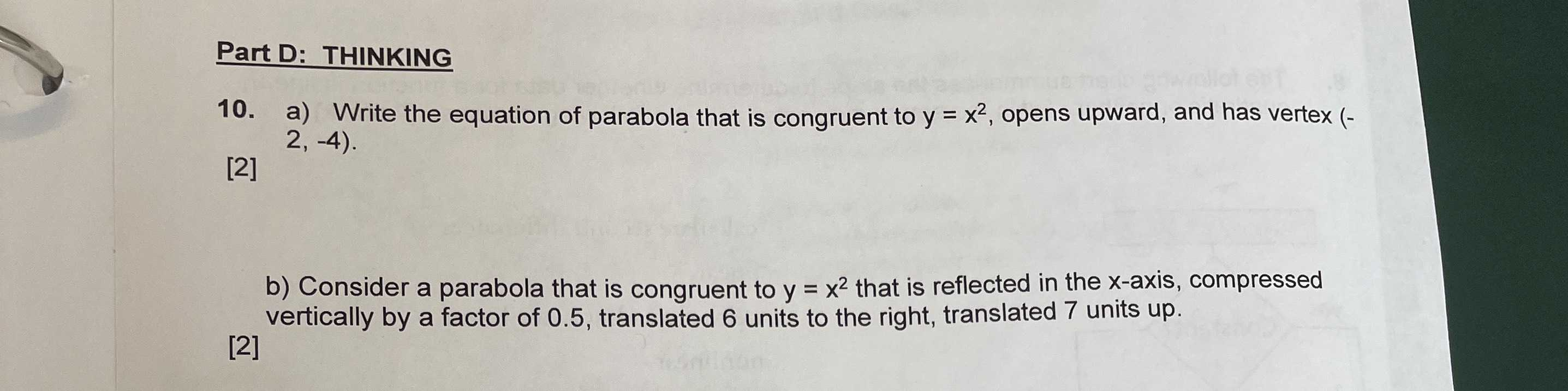### ¿Todavía tienes preguntas de matemáticas?

Pregunte a nuestros tutores expertos
Algebra
Pregunta10. a) Write the equation of parabola that is congruent to $$y = x ^ { 2 }$$ , opens upward, and has vertex (- $$2 , - 4 )$$. b) Consider a parabola that is congruent to $$y = x ^ { 2 }$$ that is reflected in the $$x$$ -axis, compressed vertically by a factor of $$0.5$$ , translated $$6$$ units to the right, translated $$7$$ units up.

a) $$(y+ 4) = (x+ 2)^2$$ , b) $$\frac{y}{2} = - (x- 6)^2+ 7$$